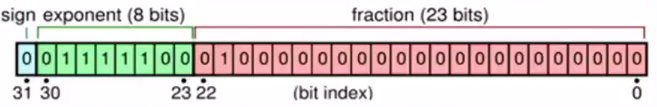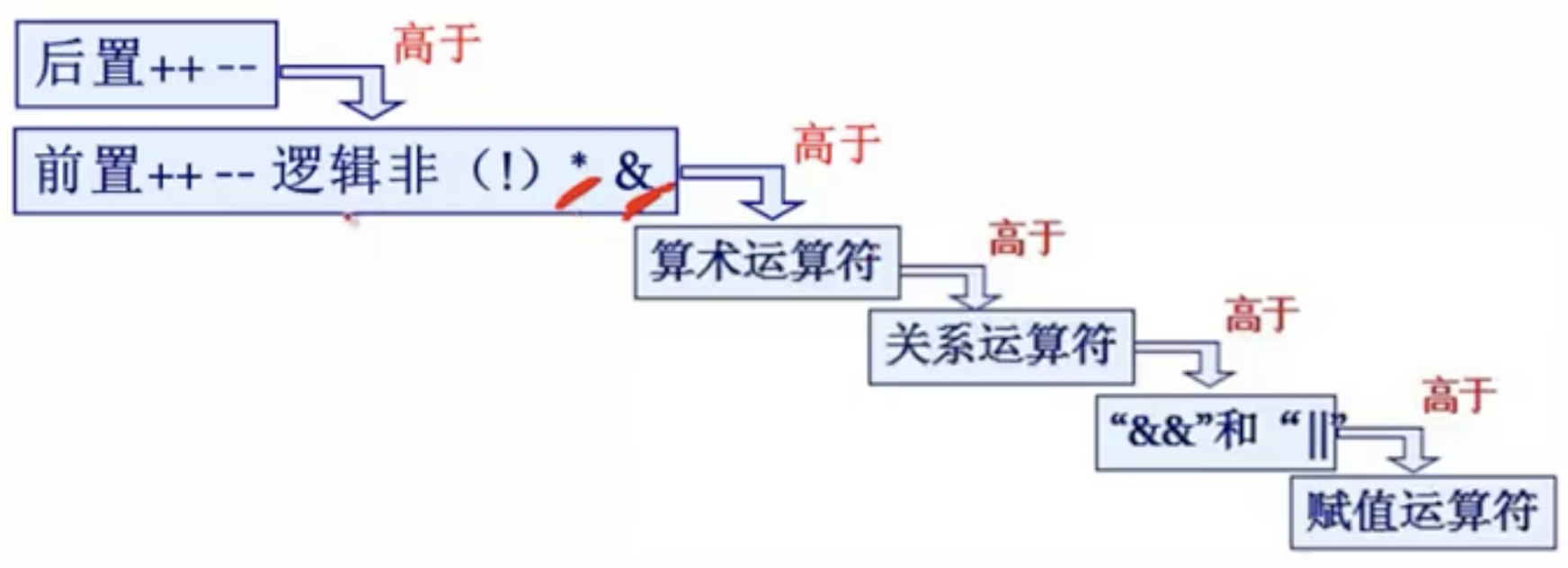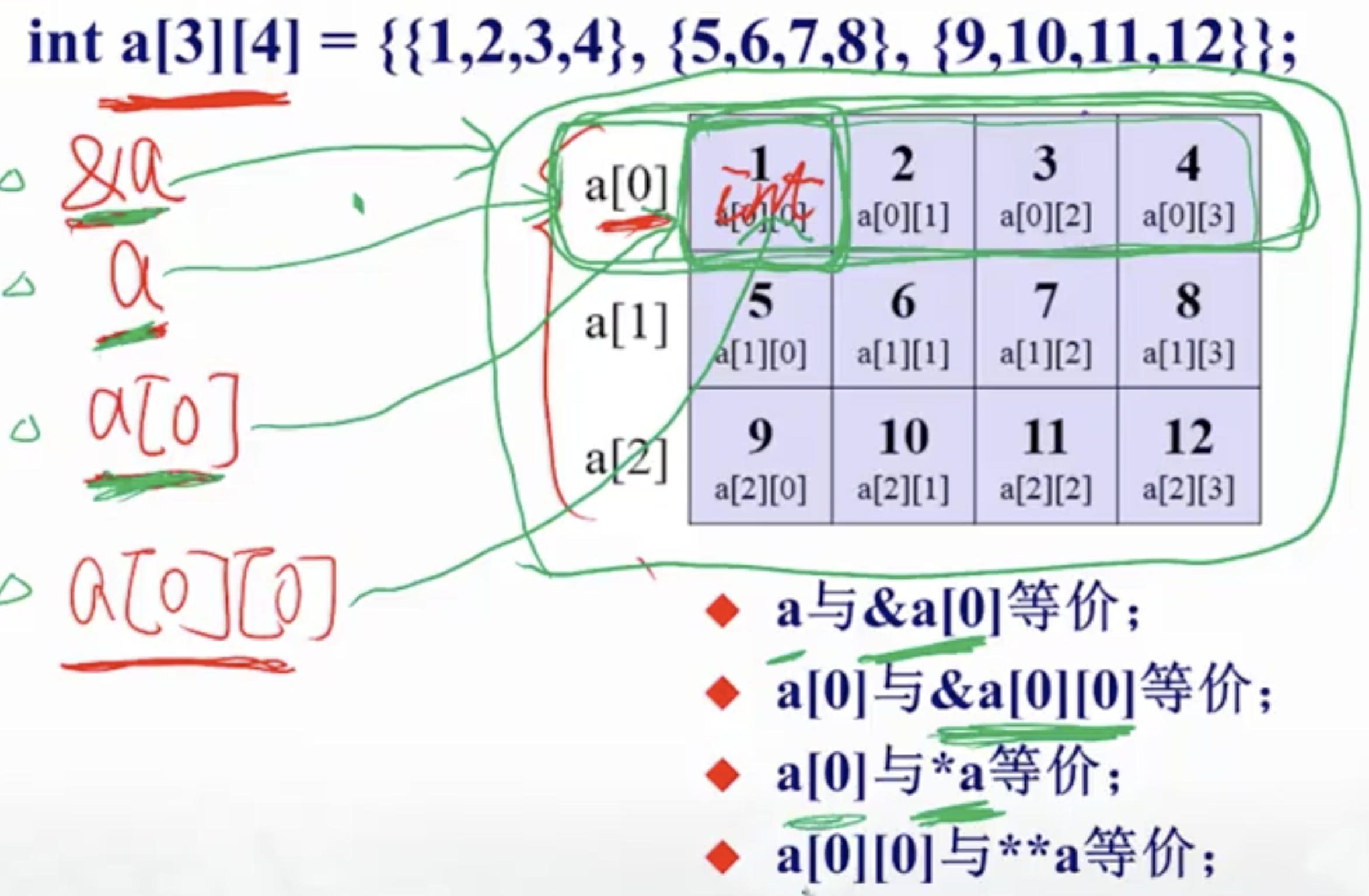# C语言语法

## C

### C语言版本

• K&R C
• 1978年，Kernighan和Ritchie的《The C Programmign Language》第一版出版，简称K&R C
• ANSI C 和 ISO C
• 1989年，C语言被标准化，对K&R C进行了扩展，包括了一些新特性，规定了一套标准函数库
• ISO成立WG14工作小组规定国际标准的C语言。
• C99
• ANSI标准化后，WG14小组继续改进C语言，1999年出版ISO9899:1999，即C99
• C11
• 2011年12月8日，ISO正式公布C语言新标准草案：ISO/IEC9899:2011, 即C11
• 标准的问题
• C语言规范定义得非常宽泛
• long型数据长度不短于int型
• short型不长于int型
• 导致：
• 不同编译器有不同的解释
• 相同的程序在不同的平台上运行结果不同
• `int`在turboC上16位，在VC上32位
• `++` ,`--`的解释不一致
• 对浮点计算的精度不同等

### 变量命名

• 匈牙利命名法
• 以一个或多个小写字母开头，来指定数据类型
• 其后是一个或多个第一个字母大写的单词，指出变量用途,如：
• `chGrade, nLength, bOnOff, strStudentName`
• 驼峰命名
• 一个变量的名字由一个或多个单词连接
• 第一个单词以小写字母开始
• 后面单词的首字母大写
• `myFirstName`

## 数据部分

### 整型

• Signed vs Unsigned:
• `unsigned int i = 123`对应内存布局为: `00000000 00000000 00000000 01111011`
• `signed int i = -123`对应的内存布局为: `11111111 11111111 11111111 10000101`
• 对于有符号数，第一个bit用来表示符号位,负数用1表示，正数用0
• 如果是`int`，那么第一个bit表示符号，实际所代表的数值用剩余31位表示
• 如果是`unsigned int`，则没有符号位，可以用32位表示每个数
• 无符号数在内存中以原码的形式存储，有符号数以补码的形式存储，计算方式为
• 无符号数取反+1
• 例如：求`-1`的二进制表示
1. 先确定符号位为`1`
2. 求出`1`的原码：`10000000 00000000 00000000 00000001`
3. 对原码部分各位取反: `11111111 11111111 11111111 11111110`
4. `+1`: `11111111 11111111 11111111 11111111` = `0xffffffff`
• 代码表示
• 十六进制`0x`开头：`int a = 0xffffff12`
• 八进制`0`开头：`int a = 037777777605`

### 最大数

• 无符号`unsigned int`:
• 十六进制： `0xffffffff`
• 十进制：`4294967295`大约42亿
• 有符号`int`:
• 十六进制 `0x7fffffff`
• 十进制：`2^31-1``INT_MAX=2147483647`大约21亿
• 二进制：`01111111 11111111 11111111 11111111`

### 最小数

• 无符号`unsigned int`:
• 十六进制： `0x000000`
• 十进制：`0`
• 有符号:
• 十六进制：`0x7fffff`(最大有符号数) + `1`
• 二进制： `10000000 00000000 00000000 00000000`（最小有符号数）
• C语言规定，当最高位是1，其它位是0时，最高位既表示负号，也表示正数最高位1

• 十进制：`-2^31``INT_MIN = -2147483648`

### 浮点型

• float : 32bit 精度7位
• double: 64bit 精度15位
• long double: 64bit 精度15位
• 浮点型的内存布局
• 第一位为符号位
• 7位指数位：最多表示2的127次方，最多表示10的38幂
• 24位二进制小数位：最多表示2的24次幂`````` 	float example = 97.148;
char binary[sizeof(float)];
memcpy(binary, &example, sizeof(float));
std::cout << "\n 32-bit float 97.148:  " << "\n";
for (int i = 0; i < sizeof(float); ++i) {
std::cout << std::bitset<sizeof(char)*8 >(binary[i]);
}
``````

我们可以通过上面代码查看任意浮点数的二进制表示如下，对照上面规则，可以看到`97.148``97.1485945`的差别极大，而`97.1485945``97.148594576678755667`二进制相同，说明后者使用32bit已经表示不下，多余位数被截断。

``` 32-bit float 97.148: 11000111010010111100001001000010 32-bit float 97.1485945: 00010101010011001100001001000010

32-bit float 97.148594576678755667:
``````00010101010011001100001001000010
```
``````
• 浮点数使用须知
• 避免将一个很大数和和一个很小数相加或相减，否则会“丢失”小的数
``````  float a = 123456.789e5
float b = a + 20
cout <<b<<endl
//由于a是float型，它可写为：`1.23456789e10`,由于它的精度只有7位，因此实际上只能精确到`1.2345678e10`,所以b的值会计算错误
``````

### Const和指针

• 指向常量的指针
• 不可以通过常量指针修改其指向的内容
• 不可以将常量指针赋值给非常量指针，但可以进行强制类型转换
``````  int n = 100;
int m = 101;
const int* p1 = &n;
int *p2 = &m;
//*p1 = 100; //compile error!
p1 = p2; //ok
printf("%d\n",*p1 ); //101
p2 = p1; //compile error!
p2 = (int* )p1; //ok，进行强制类型转换
``````
• 指针常量
• 定义：指针本身是常量，定义的时候要初始化，初始化完成后指针的值（即某对象的地址）不可修改（地址锁定）
• 表示：把`*`放在`const`之前，即`int *const p = &i;`
• 含义：
• 指针常量的值（地址）所指向的内容可以修改，但指针常量自身的值不可以被修改
``````  int err=0;
int *const pErr = &err;
*pErr = 0; //正确，修改pErr所指向的对象
const double pi = 3.1415926
const double const* pop = &pi; //pip是指向常量对象的常量指针
*pip = 2.72 //错误
``````

### 引用

• 定义引用时就初始化其值
• 初始化后，它就一直引用该变量，不会再引用别的变量了。
• 引用只能引用变量，不能引用常量和表达式
``````int n = 7;
int &r = n;
r = 4;
cout << r; //4
cout << n; //4
n = 5;
cout << r; //5

``````
• 引用做参数：`swap(int &a, int &b)`
• 引用作为函数的返回值：
``````int n=40;
int& setValue()

int main(){
setValue()=40;
cout<<n; //40
return 0;
}
``````
• const引用：
``````int n=100;
const int& r = n;
r = 200; //compiler error
n = 300; //fine
``````

### Static 变量

• 普通局部变量定义：`int a = 0;`实际上是`auto int a = 0;`,`auto`可以省略

## 运算部分

### 赋值运算

• 将长数赋值给短数
• 例如，将long型赋值给short型：
``````  int main()
{
long int long_i = 0x2AAAAAAA;
short short_j = long_i;

//long_i=00101010 10101010 10101010 10101010,会将低16bit赋值给short_j
//即10101010 10101010
//由于short_j是有符号数，那么第一位为1时为负数，即-21846
}
``````
• 将短数赋值给长数
• 将小数赋值给大数对于有符号数的规则为：
• 若小数的高位为1，则大数的高位补1
• 若小数的高位为0，则大数的高位补0
• 有符号数和无符号数互相赋值
• 不考虑符号位

### 运算符

• 运算符优先级：

• 逗号运算符
• 运算符优先级别最低
• 将两个表达式连接起来
• `exp1`,`exp2`,`exp3`,…`expn`
• 先求`exp1`再求`exp2`,…,再求`expn`，整个表达式的值为表达式`n`的值,例如:`a = 3*5, a*4;`展开为`a=15,a*4`，结果为`60`
• 考虑下面两个式子，x的值分别为多少？
• `x=(a=3,6*3)` x=18
• `x=a=3,6*3` x=3

### 位运算

• C语言中的位运算有
• `&`
• `|`
• `^` 异或，双目运算(需要两个bit参与运算)
• `~`
• `<<`，左移
• 高位左移后溢出，舍弃不起作用
• 例如`a=15`，即`00001111`,左移2位得`00111100`,即十进制数60 ： `a = a<<2`
• 左移1位相当于该数乘以2，左移两位相当于该数乘以2的平方
• 只适用于高位溢出的舍弃bit不包含1的情况
• `>>` ，右移
• 无符号数，低位移除舍弃，高位补0
• 有符号数
• 若原来的符号位为0，则左边移入0
• 若原来的符号位位1，则左移移入0还是1，由操作系统决定
• 若移入0，称为逻辑右移，或简单右移
• 若移入1，称为算术右移
• 常用的位运算
• 使特定位翻转
• 例如使`01111010`低4位翻转，可将其与`00001111`进行`^`运算，得到`01110101`
• 使特定位保持不变
• `0`进行`^`
• 互换两个数的值，而不必使用临时变量
• 例如`a=3,b=4`，交换`a,b`可用:
``````  a = a^b;
b = b^a;
a = a^b;
``````

## 控制语句

### For

• for语句的定义
``````for(expr1; expr2; expr3)
{
//语句
}
``````

• 先执行`expr1`
• 判断`expr2`是否为true，如果是true执行语句，如果是false则跳出
• 当语句执行完后执行`expr3`
• 执行完`expr3`后重新执行`expr1`

### goto

• 无条件转向语句
• 它的一般形式为:
• `goto 标识符`

## 数组

### 一维数组

• 定义数组:
``````float sheep;
int a2001;

``````

**数组大小不能为变量,可以为符号常量

• 数组初始化

• `a = {1,2,3,4};`
• `a[ ] = {1,2,3,4};`
• `a = {1,2};`剩下的元素自动补0
• `a = {0};`初始化一个全0数组

### 二维数组

• 定义二维数组
• `a`
• 定义一个3行4列的数组
• 相当于定义3个一维数组:`a`,`a`,`a`
• 内存布局
``````a
a
a
a
a
...
a
a
...
a
``````
• 初始化
• `a = { {1,2,3,4}, {5,6,7,8}, {9,10,11,12} };`
• `a = { 1,2,3,4, 5,6,7,8, 9,10,11,12}`省略里面的括号
• `a[] = { 1,2,3,4, 5,6,7,8, 9,10,11,12 }`
• `a[] = { {1},{0,6},{0,0,11} }`缺的元素补0
• `a = { 0 }`
• 访问
``````
for (int i=0; i<3; i++)
{
for(int j=0; j<4; j++)
{
cout << setw(3) << a[i][j]
}
cout << endl;
}

``````

### 数组的应用

• 桶排序
• 使用下标做统计
• 一维二维数组
• 寻找素数
``````#include<iostream>
#include<cmath>

using namespace std;
int main(){

int sum=0, a={0};
for(int i=2; i<sqrt(100.0); i++){

sum = i;

if(a[sum] == 0 ){

while(sum < 100){
sum +=i ;
if(sum < 100){
a[sum] = 1; //数组标记出能被i以及i的倍数整除的元素
}
}
}
}
for(int i=2; i<100; i++){
if(a[i] == 0){
cout<<i<<"";//输出所有未被标记的即为素数
}
}

return 0;
}

``````

## 字符串

### 字符数组

• 定义
• `char a = {'a','b','c','d'}`
• `char a = {'a','b'}` 剩下的元素被初始化为`\0`
• `char a[ ] = {'C','h','i','n','a'}`
• `char a[ ] = "China"`使用这种方式初始化，数组末尾会自动多一个`\0`
• `char a = "China"`这种初始化方法是错误的
• 赋值
• 只可以： 在数组定义并且初始化时:`char c = "China"`
• 不可以：不能用赋值语句将一个字符串常量或字符数组直接赋值给另一个字符数组
• `str1[] = "China"` 错误
• `str1 = "China"` 错误
• `str2=str1` 错误
• 正确的赋值方式:
``````  char str1 = "abc", str2;
while(str1[i] != '\0')
{
str2[i] = str1[i];
i++;
}
str2 = '\0';
``````
• 输出/输出
• 使用`cout`输出字符数组，要确保数组以`\0`结尾
• 使用`cin`输入字符数组时，默认空格和回车作为字符串间断
• 二位数组
``````char weekday={"Sunday","Monday","Tuesday","Wednesday","Thursday","Firday",""Saturday}.
for(int i=0;i<7;i++){
cout<<weekday[i]<<endl;
}
``````

### 常用字符串函数

• 字符串长度`strlen`，要求字符串以`\0`结尾
• `int i = strlen(len)`
``````  //strlen的实现
int strlen(char d[]){
int i=0;
while(d[i] != '\0'){
i++;
}
return i
}
``````
• 比较字符串`strcmp`，如果`p1=p2`返回`0``p1>p2`返回一个正值，`p1<p2`返回一个负值
• `bool v = strcmp(str1,str2)`
``````  //strcmp实现
int strcmp(char *s1, char *s2) {
int i;
for (i = 0; s1[i] == s2[i]; ++i ) {
if(s1[i] == '\0' && s2[i] == ‘\0')
return 0; // 两个字符串相等
}
// 不等, 比较第一个不同的字符
return (s1[i]-s2[i]) / abs(s1[i]-s2[i]);
}
``````
• 追加字符串`strcat`
• `strcat(p1,p2); //将p2追加到p1后面，返回p1`
• 拷贝字符串`strcpy`
• `strcpy(p1,p2);//将p2拷贝到p1，返回p1`
``````  //strcpy的实现
char* strcpy(char* dst, char* src){
int i=0;
while(src[i] != '\0'){
dst[i] = src[i];
i++;
}
dst[i] = '\0';
return dst;
}
``````
• 切分字符串 `strtok`
`````` char input[] = "A bird came down the walk";
char *token = strtok(input, " ");
while(token) {
puts(token);
token = strtok(NULL, " ");//如果第一个参数为null，表示在前一次的位置继续向后查找
}
``````

## 函数

### 函数的声明

• 函数原型：由函数的返回类型，函数名，以及参数表构成的一个符号串，其中参数可以不写名字，例如
``````bool checkPrime(int)
``````
• 函数的原型又称为函数的signature
• C语言中函数的声明就是使用函数的原型

### 函数的执行过程

``````
float max(float a, float b)
{
return a>b?a:b;
}

int main()
{
int m=3, n=4;
float result = 0;

result = max(m,n);

cout << result;

return 0;

}

``````
1. 调用max函数时，开辟新的stack
2. 将参数m,n传递过去，max函数接收到的参数a,b是m,n的值，但是m,n有各自的存储空间
3. max执行完成后释放stack
4. 接收函数的返回值
5. 恢复现场，从断点处执行

### 参数传递

• 实参与形参具有不同的存储单元，实参与形参变量的数据传递是“值传递”
• 函数调用时，系统给形参在函数的stack上分配空间，并将实参的值传递给形参
• 数组名做函数参数
``````void change(int a[]){
//...
}
``````
• 可变参数
``````#include <stdarg.h>  //包含头文件
int sum(int num, ... ){
va_list arg_list;
va_start(arg_list,num);//最后一个参数提示类型
int sum = 0;
for(int i=0;i<num;i++){
sum += va_arg(arg_list, int); //根据type来提取参数
}
va_end(arg_list); //成对出现
return sum;
}
void foo(char *fmt, ...)
{
va_list ap;
int d;
char c, *s;

va_start(ap, fmt);
while (*fmt) //注意参数顺序要和`fmt`的匹配规则一致
switch (*fmt++) {
case 's':              /* string */
s = va_arg(ap, char *);
printf("string %s\n", s);
break;
case 'd':              /* int */
d = va_arg(ap, int);
printf("int %d\n", d);
break;
case 'c':              /* char */
/* need a cast here since va_arg only
takes fully promoted types */
c = (char) va_arg(ap, int);
printf("char %c\n", c);
break;
}
va_end(ap);
}

int main(){
printf("sum :%d\n",sum(3,1,2,3));
foo("%s,%d,%c","hello",123,'x');
return 0;
}
``````

### 递归

• 递归调用跟函数的嵌套调用没有区别，开辟新的空间
• 用递归解决具有递推关系的问题
• 关注点放在求解目标上
• 找到第`n`次和`n-1`次之间的关系
• 确定第一次的返回结果
• 递归用来描述重复性的动作，代替循环
• 连续发生的动作是什么 -> 确定递归函数，入参
• 和前一次动作之间的关系 -> 通项公式
• 边界条件是什么 -> 递归终止的边界
• 进行”自动分析“
• 先假设有一个函数能给出答案
• 在利用这个函数的前提下，分析如何解决问题
• 搞清楚最简单的情况下答案是什么
• 常见的递归问题
• 打印二进制
``````  void convert(int x){
if((x/2)!=0){
convert(x/2);
cout<<x%2;
}else{
cout<<x;
}
}
``````
• 汉诺塔问题
``````  /*两种解法：
1. 可以先枚举—>递推->得到通项公式
2. 简化问题:
(1)移动2个 = 两次移动1个的次数 + 移动一次底座
(2)移动3个 = 两次移动2个的次数 + 移动一次底座
(3)移动n个 = 两次移动(n-1)个的次数 + 移动一次底座
*/
int  hanno(int n)
{
if (n == 1){
return 1;
}
return 2*hanno(n-1)+1;
}
``````
• 逆波兰表达式
``````  /*
逆波兰表达式是一种把运算符前置的算术表达式
如：2+3 的逆波兰表示法为 + 2 3
如：(2+3)*4 的逆波兰表示法为 x + 2 3 4
输入：x + 11.0 12.0 + 24.0 35.0
输出：1357.0
*/

//伪代码
void reverse (deque<string> s){
string token = s.front();
s.pop_front();
if (token == "+")
{
return notation(s) + notation(s);
}
else if (token == "-")
{
return notation(s) - notation(s);
}
else if (token == "x")
{
return notation(s) * notation(s);
}
else if (token == "/")
{
return notation(s) / notation(s);
}
else
{
return stof(token);
}
}
``````

## 指针

### 指针与指针变量

• 某个变量的地址称为”指向该变量的指针”，注意:“地址” == “指针”
• `0x0012ff78`这个地址就是它指向变量的指针
• 例如，`http://www.nasa.gov/assets/images/content/166502.jpg`是一幅图片的指针
• 存放地址的变量称为指针变量

• 指针变量也有自己的地址

• 定义:`int *pointer`
• `pointer`是变量名
• `*`代表变量的类型是指针
• `int`表示指针变量的基类型，即指针变量指向变量的类型
• 赋值，表达式:`&E`，取变量`E`的地址
``````  int *pointer;
int c=100;
pointer = &c;
``````
• 访问，表达式：`*E`，如果`E`是指针，返回`E`所指向的内容
``````  int d = *pointer;
*pointer = 49;
``````
• 例子
``````#include <iostream>
using namespace std;

int main(){

int count = 18;
int *pointer = &count;
*pointer = 58;
cout<<count<<endl; //58
cout<<pointer<<endl; // 0x7ffee67631d8
cout<<&count<<endl; // 0x7ffee67631d8
cout<<*pointer<<endl; // 58
cout<<&pointer<<endl; //0x7ffee67631d0

return 0;
}
``````
• `&``*`的优先级
• `*&a = *(&)a`
• `&*a = &(*)a`
• `(*a)++ != *(a++)`### 数组与指针

• 数组名代表数组元素的首地址
• 数组名相当于指向数组第一个元素的指针
• 对数组名取地址`&a`的值等同于数组第一个元素的地址`a`，但是含义不同
• 返回基类型为数组的指针，意思是，当`&a+1`是，指针跨过的是整个数组长度和`a+1`不同
``````  int a = {1,3,4,6};
cout<<a<<end; 			//0x0028f7c4
cout<<&a<<endl;			//0x0028f7c4
cout<<a+1<<endl;			//0x0028f7c4 + 4  = 0x0028f7c8
cout<<&a+1<<endl;		//0x0028f7c4 + 16 = 0x0028f7d4
cout<<*(&a)<<endl;		//0x0028f7c4
cout<<*(&a)+1<<endl;	//0x0028f7c8
``````
• 指向数组的指针：
• `int a; int *p; p=a;`p为指向数组的指针
• `char a; char* p; p = a;`p为指向字符串的指针
• 数组名做函数参数
• C++编译器将形参数组名作为指针变量来处理
``````int sum(int array[], int n){
for(int i=0;i<n; i++){
array[i]++;
}
}

int main(){
int a={1,2,3,4,5,6,7,8,9,10};
sum(a,10);
}
``````
• 二维数组
• 理解二维数组• 索引二维数组
``````  int a = { {1,3,5,7}, {9,11,13,15}, {17,19,21,23} };
int (*p) = a;
for(int i=0;i<3;i++){
for(int j=0;j<4;j++){
cout<<*(*(p+i)+j)<<endl;
// cout<<p[i][j]<<endl;
}
}
``````

假设有数组`a`，是一个三行四列的数组，如何使用指针来索引？

1. `p=a`开始
• `a`相当于指向`a`第一个元素的指针，所谓第一个元素即是第一个子数组`{1,3,5,7}`，所以`a`是一个第一个组数组的首地址
• 指针`p`的基类型应该与`a`相同，即“包含四个整形元素的一维数组”
• 定义：`int (*p)``p`指向`a`的第一个子数组
2. 分析`*(*(p+i)+j)`
• `p+i`是第`i`个子数组的地址，等价于`&a[i]`
• `*(p+i)`等价于`a[i]`
• `*(p+i)+j`等价于`a[i]+j`等价于`&a[i][j]`
• `*(*(p+i)+j)`等价于`a[i][j]`等价于`p[i][j]`
• 三条规律
• 数组名相当于指向数组第一个元素的指针
• `&E`相当于把`E`的管辖范围升了一级
• `*E`相当于把`E`的管辖范围降了一级

### 指针与函数

#### const指针

• 定义：`const int* p`;
• `const`的意思是指向符号常量的指针，即指针所指向的内容为常量
• 定义`const`指针时，需要直接初始化其值
``````{
const int a = 78; const int b = 28; int c = 18;
const int *pi = &a;
int *p2 = pi; //error，不能将const指针赋值给非const指针
int p2 = (int* )pi; //可以将const指针强制类型转化为非const指针
*pi = 100; //error,*p不能被赋值
pi = &b; //可以，pi本身的值可以修改
*pi = 68; //error,*p不能被赋值
pi = &c; *pi = 100; //error,*p不能被赋值

}
``````
• 数组名函数参数时，为了防止被函数修改，可以加上const限定符
``````int sum(const int array[], int n){
//不能对array进行修改
}

``````
• 指针做函数返回值
``````int *get(int arr[], int n, int m){
int *pt;
pt = *(arr + n - 1) + m-1;
return pt; //返回二维数组中arr[n][m]的地址
}
int main(){
int a={ {1,2,3,4},{5,6,7,8},{9,10,11,12},{13,14,15,16} };
int *p;
p = get(a,2,3);
cout<<*p<<endl;
}
``````
• 函数指针
• 格式：`类型名(*指针变量名*)(参数类型1，参数类型2，...)`
• `int (*pf)(int a, int b)`
• 使用：
• 将函数指针做参数传递，需要`typedef`一个类型
• 函数指针可以实现多态

## Struct

### 定义与赋值

• 定义结构体: - 语法：`struct STUDENT {...};` , STUDENT`表示结构体类型，类似`int,char`等

• 使用结构体定义变量
• `struct` + 结构体类型名 + 变量名:
• `struct STUDENT stu1, stu2;`
• 也可以省略`struct`关键字 `STUDENT mike = {123, "mike"};`
• 使用`typedef`:
• `typedef struct _STUDENT{...}STUDENT;`
• 其中`_STUDENT`叫做struct tag，可以省略
• 使用:`STUDENT stu1,stu2`
• 在声明类型的同时定义变量:
``````  struct Person {
char *name;
int age;
int height;
int weight;
}xt1,xt2;
``````
• 结构体之间的拷贝
• 结构体做函数参数，赋值会产生内存拷贝
• 结构体做函数返回值，也会产生内存拷贝
``````struct student x1 = {1,2};
struct student x2;
x2 = x1;
//x2中的值相当于x1中的值的copy，同理，结构体变量做函数参数和返回值也是copy的
``````

### 结构体的大小

• 结构体的大小涉及到内存对齐
``````#include <stdio.h>
using namespace std;

struct P
{
int num;
char name;
};

union T{
int num;
char name;
};

int main()
{
printf("size of int: %lu\n",sizeof(int)); //4
printf("size of struct: %lu\n",sizeof(P)); // 16
printf("size of uion: %lu\n",sizeof(T)); //12

return 0;
}

``````
• `union`的大小也涉及内存对齐

In a union, at most one of the data members can be active at any time, that is, the value of at most one of the data members can be stored in a union at any time. [Note: one special guarantee is made in order to simplify the use of unions: If a POD-union contains several POD-structs that share a common initial sequence (9.2), and if an object of this POD-union type contains one of the POD-structs, it is permitted to inspect the common initial sequence of any of POD-struct members; see 9.2. ] The size of a union is sufficient to contain the largest of its data members. Each data member is allocated as if it were the sole member of a struct.

### 结构体指针与数组

• 结构体指针
``````struct Person {
int id_num;
char name;
};
int main(){
Person mike = {123,{'J','o'}};
Person *one = &mike;
cout<<*(one).id_num<<endl;
cout<<one->id_num<<endl;
}
``````
• 结构体数组
• 数组名相当于指向数组第一个元素的指针
• 指向元素的之后怎++，则跨过一整个结构体

### 应用

• 链表：
• 链表头：指向第一个链表节点的指针
• 链表节点：链表中的每一个元素，包括：
• 当前节点的数据
• 下一个节点的地址
• 链表尾部：不在指向其他节点，其下一个节点的指针为NULL，表示链表结束
``````struct student{
int id;
student* next;
};
``````

• 《C程序设计》